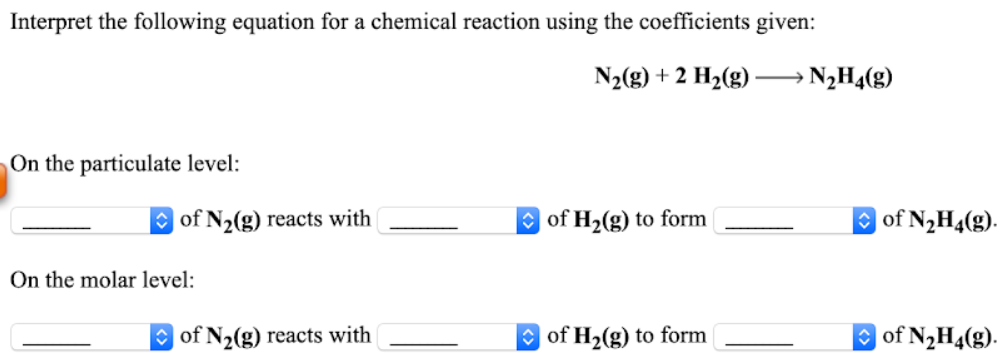# Interpret the following equation for a chemical reaction using the coefficients given: N2(g) + 2H2(g) → N2H4(g) On the particulate level: _____ of N2(g) reacts with _____ of H2(g) to form _____ of N2H4(g). On the molar level: _____ of N2(g) reacts with _____ of H2(g) to form _____ of N2H4(g).